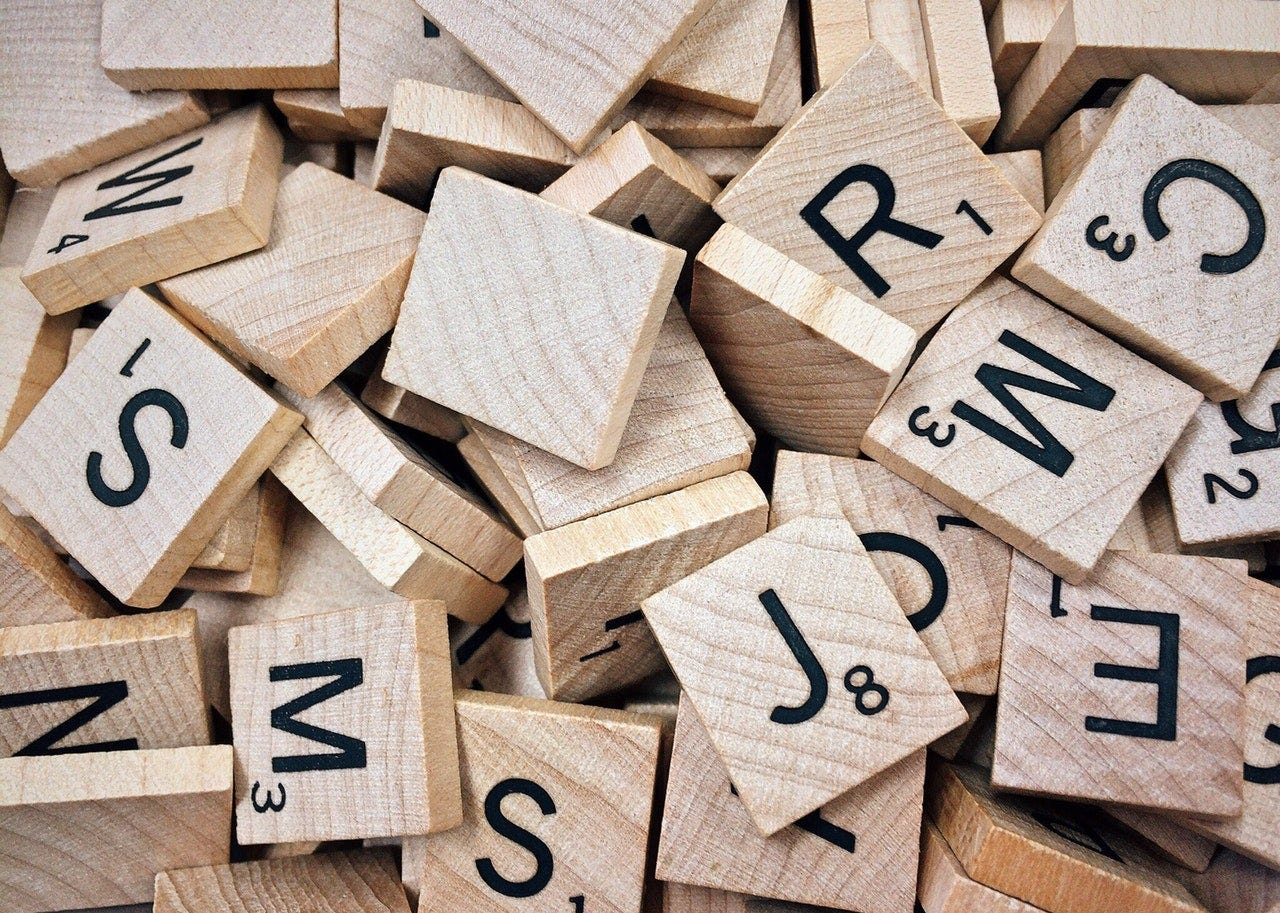Photo by Pixabay from Pexels

(Tutorial) Unleashing the power of examples in learning regex. Part 1 with example on email extraction (with python & c++ code)

Let’s start with the basics.

What’s REGEX?

Regex is the tool to find specified matches in string like: e-mails, names, cities, syntax errors, tickers etc …

To put it simply when you want to extract specified words (in our example emails) from the below text — regext is your friend!

`This is an example string to show you that this email example@example.com can be easily extracted from such text.`

Regex is used everywhere and in general its fundamentals are mostly the same across all programming languages.

Regex is a must-have skill for any programmer, data scientists or machine learning scientist! Once you get to know the basics and see a few examples you will be astonished how easy it is.

Let’s start with the example — finding e-mails in text:

As a programmer it is very common to get a task of finding and extracting some information from a website or a document. In this example, let’s focus on extracting e-mails.

For example:

Let’s imagine you are assigned with a very common task to extract all e-mails from a string. In other hand, to create a class that takes as input a raw string and outputs all e-mails within a string.

How to do it?

Here is the solution:

`‘[\w.-]+@[\w.-]+.\w+’`

OK, let’s go with a thought process.

We need to start how e-mails look like, what are potential combinations of e-mails.

We can have:

The first thing, the most important one is WHAT’S COMMON IN ALL EMAIL ADDRESSES….

This is @ — simply all e-mails contain @. But before and after @ there may be any combination of words or special characters. So how to find them all?

Our goal is to find every combination of words before and after @. In regex to specify any word combination we need to use a special symbol — \w.

— \w is for ASCII letter(e.g. a b c), digit(e.g. 1 2 3 or underscore (e.g. _).

But in email we can also have a dot ( . ) so in regex dot is a dot — . (in fact dot represents any character).

And also we can have a dash ( — ) so in regex dash is a dash ( - )

So how to merge \w, dot (.) and dash (-) together? Simply using a square brackets [] like →

`[\w.-].`

We also should add the plus symbol at the end to tell regex engine that this combination of any symbols may repeat many times, hence our first part of the regex looks now:

`‘[\w.-]+’`

Now we add @ as a common pattern for all emails.

`‘[\w.-]+@’`

and now, we need to add the same pattern after @:

`‘[\w.-]+@[\w.-]+’`

all email addresses end with .com / .pl / .eu / .xxx, so we should also add it:

`‘[\w.-]+@[\w.-]+.\w+’`

let’s see a complete working python code:

`import reexample_string = ‘This is example string with example@example.com mail and with example-example@example.com.’all_emails = re.findall(r’[\w.-]+@[\w.-]+.\w+’, example_string)print(all_emails)`

now let’s see a complete working c++ code:

`#include <iostream>#include <regex>#include<string.h>using namespace std;int main(){string example_string = “This is example e@example.com string with example@example.com mail and with exampleexample@example.com kuku@example.com. Some other text.”;regex rexp(“[\\w.-]+@[\\w.-]+.\\w+ “);smatch m;int i = 1;while (regex_search(example_string, m, rexp)) {cout << “\nMatched string is “ << m.str(0) << endl<< “and it is found at position “<< m.position(0) << endl;i++;example_string = m.suffix().str();}cout << “Maslo “ << example_string << endl;return 0;}`

The difference between python and c++ is an additional white space in c++ at the end of the regex sentence. why? — python as interpreter language allows for that while c++ is more explicit.

All the best!